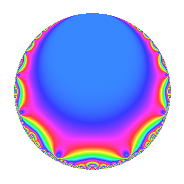# Properties

 Label 448.2.a.gLevel 448 Weight 2 Character orbit 448.a Self dual yes Analytic conductor 3.577 Analytic rank 0 Dimension 1 CM no Inner twists 1

# Related objects

## Newspace parameters

 Level: $$N$$ = $$448 = 2^{6} \cdot 7$$ Weight: $$k$$ = $$2$$ Character orbit: $$[\chi]$$ = 448.a (trivial)

## Newform invariants

 Self dual: yes Analytic conductor: $$3.57729801055$$ Analytic rank: $$0$$ Dimension: $$1$$ Coefficient field: $$\mathbb{Q}$$ Coefficient ring: $$\mathbb{Z}$$ Coefficient ring index: $$1$$ Twist minimal: no (minimal twist has level 14) Fricke sign: $$-1$$ Sato-Tate group: $\mathrm{SU}(2)$

## $q$-expansion

 $$f(q)$$ $$=$$ $$q + 2q^{3} + q^{7} + q^{9} + O(q^{10})$$ $$q + 2q^{3} + q^{7} + q^{9} + 4q^{13} + 6q^{17} - 2q^{19} + 2q^{21} - 5q^{25} - 4q^{27} + 6q^{29} - 4q^{31} - 2q^{37} + 8q^{39} + 6q^{41} - 8q^{43} - 12q^{47} + q^{49} + 12q^{51} - 6q^{53} - 4q^{57} + 6q^{59} - 8q^{61} + q^{63} + 4q^{67} + 2q^{73} - 10q^{75} + 8q^{79} - 11q^{81} + 6q^{83} + 12q^{87} - 6q^{89} + 4q^{91} - 8q^{93} - 10q^{97} + O(q^{100})$$

## Embeddings

For each embedding $$\iota_m$$ of the coefficient field, the values $$\iota_m(a_n)$$ are shown below.

For more information on an embedded modular form you can click on its label.

Label $$\iota_m(\nu)$$ $$a_{2}$$ $$a_{3}$$ $$a_{4}$$ $$a_{5}$$ $$a_{6}$$ $$a_{7}$$ $$a_{8}$$ $$a_{9}$$ $$a_{10}$$
1.1
 0
0 2.00000 0 0 0 1.00000 0 1.00000 0
 $$n$$: e.g. 2-40 or 990-1000 Significant digits: Format: Complex embeddings Normalized embeddings Satake parameters Satake angles

## Inner twists

This newform does not admit any (nontrivial) inner twists.

## Twists

By twisting character orbit
Char Parity Ord Mult Type Twist Min Dim
1.a even 1 1 trivial 448.2.a.g 1
3.b odd 2 1 4032.2.a.w 1
4.b odd 2 1 448.2.a.a 1
7.b odd 2 1 3136.2.a.e 1
8.b even 2 1 14.2.a.a 1
8.d odd 2 1 112.2.a.c 1
12.b even 2 1 4032.2.a.r 1
16.e even 4 2 1792.2.b.c 2
16.f odd 4 2 1792.2.b.g 2
24.f even 2 1 1008.2.a.h 1
24.h odd 2 1 126.2.a.b 1
28.d even 2 1 3136.2.a.z 1
40.e odd 2 1 2800.2.a.g 1
40.f even 2 1 350.2.a.f 1
40.i odd 4 2 350.2.c.d 2
40.k even 4 2 2800.2.g.h 2
56.e even 2 1 784.2.a.b 1
56.h odd 2 1 98.2.a.a 1
56.j odd 6 2 98.2.c.a 2
56.k odd 6 2 784.2.i.c 2
56.m even 6 2 784.2.i.i 2
56.p even 6 2 98.2.c.b 2
72.j odd 6 2 1134.2.f.f 2
72.n even 6 2 1134.2.f.l 2
88.b odd 2 1 1694.2.a.e 1
104.e even 2 1 2366.2.a.j 1
104.j odd 4 2 2366.2.d.b 2
120.i odd 2 1 3150.2.a.i 1
120.w even 4 2 3150.2.g.j 2
136.h even 2 1 4046.2.a.f 1
152.g odd 2 1 5054.2.a.c 1
168.e odd 2 1 7056.2.a.bd 1
168.i even 2 1 882.2.a.i 1
168.s odd 6 2 882.2.g.c 2
168.ba even 6 2 882.2.g.d 2
184.e odd 2 1 7406.2.a.a 1
280.c odd 2 1 2450.2.a.t 1
280.s even 4 2 2450.2.c.c 2

By twisted newform orbit
Twist Min Dim Char Parity Ord Mult Type
14.2.a.a 1 8.b even 2 1
98.2.a.a 1 56.h odd 2 1
98.2.c.a 2 56.j odd 6 2
98.2.c.b 2 56.p even 6 2
112.2.a.c 1 8.d odd 2 1
126.2.a.b 1 24.h odd 2 1
350.2.a.f 1 40.f even 2 1
350.2.c.d 2 40.i odd 4 2
448.2.a.a 1 4.b odd 2 1
448.2.a.g 1 1.a even 1 1 trivial
784.2.a.b 1 56.e even 2 1
784.2.i.c 2 56.k odd 6 2
784.2.i.i 2 56.m even 6 2
882.2.a.i 1 168.i even 2 1
882.2.g.c 2 168.s odd 6 2
882.2.g.d 2 168.ba even 6 2
1008.2.a.h 1 24.f even 2 1
1134.2.f.f 2 72.j odd 6 2
1134.2.f.l 2 72.n even 6 2
1694.2.a.e 1 88.b odd 2 1
1792.2.b.c 2 16.e even 4 2
1792.2.b.g 2 16.f odd 4 2
2366.2.a.j 1 104.e even 2 1
2366.2.d.b 2 104.j odd 4 2
2450.2.a.t 1 280.c odd 2 1
2450.2.c.c 2 280.s even 4 2
2800.2.a.g 1 40.e odd 2 1
2800.2.g.h 2 40.k even 4 2
3136.2.a.e 1 7.b odd 2 1
3136.2.a.z 1 28.d even 2 1
3150.2.a.i 1 120.i odd 2 1
3150.2.g.j 2 120.w even 4 2
4032.2.a.r 1 12.b even 2 1
4032.2.a.w 1 3.b odd 2 1
4046.2.a.f 1 136.h even 2 1
5054.2.a.c 1 152.g odd 2 1
7056.2.a.bd 1 168.e odd 2 1
7406.2.a.a 1 184.e odd 2 1

## Atkin-Lehner signs

$$p$$ Sign
$$2$$ $$1$$
$$7$$ $$-1$$

## Hecke kernels

This newform subspace can be constructed as the intersection of the kernels of the following linear operators acting on $$S_{2}^{\mathrm{new}}(\Gamma_0(448))$$:

 $$T_{3} - 2$$ $$T_{5}$$ $$T_{11}$$